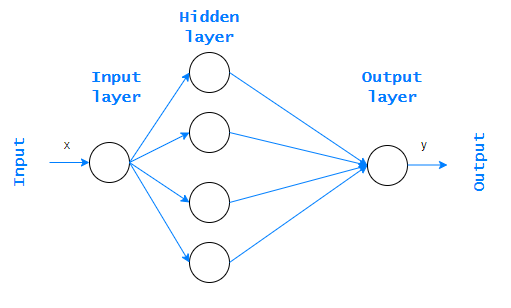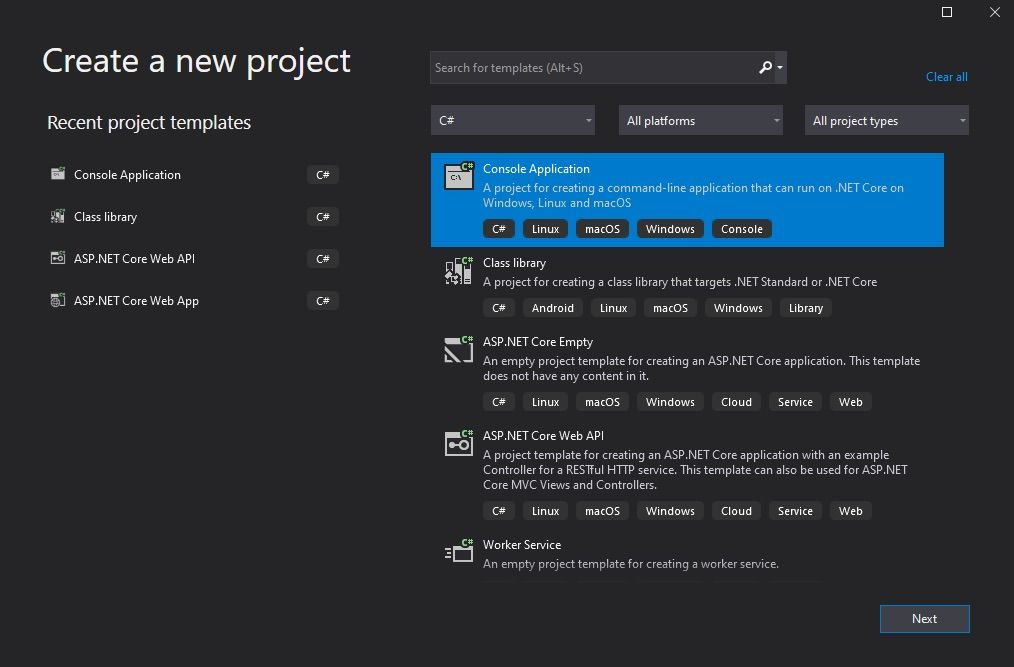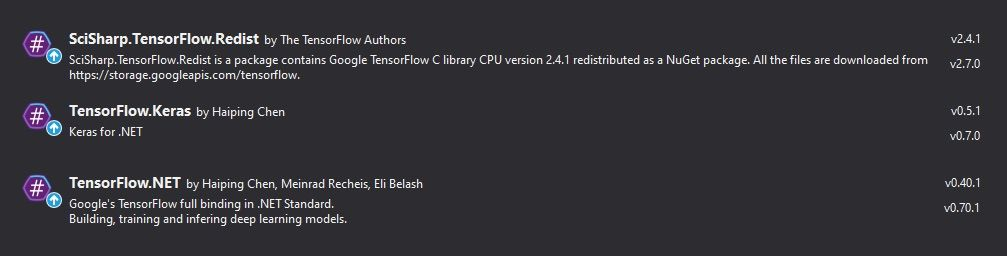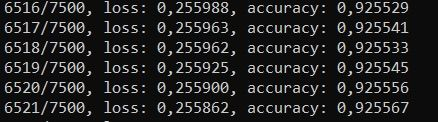Facilitating the Spread of Knowledge and Innovation in Professional Software Development

Write for InfoQ

### Topics

InfoQ Homepage Articles Building Neural Networks with TensorFlow.NET

# Building Neural Networks with TensorFlow.NET

### Key Takeaways

• TensorFlow is an open-source framework developed by Google scientists and engineers for numerical computing.
• TensorFlow.NET is a library that provides a .NET Standard binding for TensorFlow, allowing you to design neural networks in the .NET environment.
• Neural Networks may be applied wherever answers, not quantitative but qualitative, are required.
• Feedforward Neural Network (FNN) is one of the basic types of Neural Networks and is also called multi-layer perceptrons (MLP).
• The Feedforward Neural Network (FNN) algorithm returns Loss Function and Accuracy in each iteration

Building and creating Neural Networks is mainly associated with such languages/environments as Python, R, or Matlab. However, there have been some new possibilities in the last few years.

Among others, within .NET technology. Of course, you can work on Neural Networks from scratch in every language, but it is not a part of the scope of this article. In my first words, I was thinking about libraries that give us such opportunities. In this article, I will focus on one of these libraries called TensorFlow.NET.

## Neural Networks

Let me explain the general concept of Neural Networks. We will centre on the Feedforward Neural Network (FNN), which is one of the basic types of neural networks. this type of Neural Network is also called multi-layer perceptrons (MLP).

The objective of the Feedforward Neural Network is to approximate some function f*.

Neural Networks use classifiers, which are algorithms that map the input data to a specific category.

For instance, for a classifier, y = f*(x) maps the input x to the category y. MLP determines the mapping y=f(x;α) and learns the parameter values α - exactly those that provide the best approximation of the function.

Note the graphic below. It shows an MLP perceptron, which consists of one input layer, at least one hidden layer, and an output layer.

This is called a feedforward network because information always goes in one direction. It never goes backwards. It is worth mentioning that if a neural network contains two or more hidden layers, we call it the Deep Neural Network (DNN).### The main applications of Neural Networks

Artificial Neural Networks are a fundamental part of Deep Learning. They are mathematical models of biological neural networks based on the concept of artificial neurons. In artificial neural networks, an artificial neuron is treated as a computational unit that, based on a specific activation function, calculates at the output a certain value on the basis of the sum of the weighted input data.  They are scalable and comprehensive compared to other machine learning algorithms, which suit perfectly huge and complex Machine Learning tasks. Due to the specific features and unique advantages, the application area of neural networks is extensive.

We can apply them wherever not quantitative but qualitative answers are required. Quantitative data relates to any information that can be counted or measured, and to which a numerical value can be given. On the other hand, qualitative answers are descriptive in nature, expressed in language rather than numerical values. Therefore, Neural Networks include applications such as:

• price forecasting
• interpretation of biological tests
• analysis of production problems
• medical researches
• electronic circuit diagnostics
• sales forecasting

For better understanding, I will give you the following examples from real life. A certain company with business in 20 countries in the Central and Eastern European market distributes components for hydraulic and temperature control systems. The problem lies in determining the amount of stock available at each distribution point. The administrative staff has been collecting detailed data for several years by using reports on the quantity of demand and supply for a given type of product. This data shows that sometimes too much is stored, and sometimes there are shortages in supply. It was decided to use an artificial neural network to solve this problem.

In order to create the inputs of the neural network, reports from 5 years of the stores' prosperity were used. This resulted in 206 cases prepared by office workers, of which 60% were used for the learning set, 20% for verification and the remaining 20% for testing. Each case contained certain features such as weather conditions in a particular region or locations of stores, i.e., whether they are in downtown or the suburbs.

The results were not sufficiently convincing to use the created algorithm for stock management (effectiveness within 65%), but the result was three times better and closer to the truth than “classical methods” based on basic calculations. It can be concluded that the author will make another attempt to create a system taking into account more factors in order to obtain a more reliable solution.

Artificial Neural Networks are used in various branches of science, economy, and industry. Since more and more entrepreneurs and specialists are noticing their effectiveness, the number of technologies that allow for their creation and implementation into commercial systems is also growing. There are many libraries implementing the technologies I mentioned before One of them is TensorFlow.NET, which I will introduce in a moment.

## TensorFlow.NET

TensorFlow.NET is a library that provides a .NET Standard binding for TensorFlow. It allows .NET developers to design, train and implement machine learning algorithms, including neural networks. Tensorflow.NET also allows us to leverage various machine learning models and access the programming resources offered by TensorFlow.

### TensorFlow

TensorFlow is an open-source framework developed by Google scientists and engineers for numerical computing. It is composed by a set of tools for designing, training and fine-tuning neural networks.TensorFlow's flexible architecture makes it possible to deploy calculations on one or more processors (CPUs) or graphics cards (GPUs) on a personal computer, server, without re-writing code.

### Keras

Keras is another open-source library for creating neural networks. It uses TensorFlow or Theano as a backend where operations are performed. Keras aims to simplify the use of these two frameworks, where algorithms are executed and results are returned to us. We will also use Keras in our example below.

## Example usage of TensorFlow.NET

In this example, we will create FNN and use some terms related to Neural Networs such as layers, the loss function etc. I recommend checking out this article written by Matthew Stewart to understand all these terms.

First, you need to create a console application project (1) and download the necessary libraries from NuGet Packages (2).(1)(2)

At this point, you can start to implement and create the model. The first step is to create a class corresponding to your neural network. It should include the fields of the model, learning and test set. The Model class comes from TensorFlow.Keras.Engine, and NDArray is just part of NumSharp, which is the corresponding NumPy library known in the Python world.

using NumSharp;
using Tensorflow.Keras.Engine;
using Tensorflow.Keras.Layers;
using static Tensorflow.KerasApi;

namespace NeuralNetworkExample
{

public class Fnn
{
Model model;
NDArray x_train, y_train, x_test, y_test;

.....
}
}


The second step is to generate test and training sets. For this purpose, we will use the MNIST datasets from Keras. MNIST is a massive database of digits that is used to train various image processing algorithms. This dataset is loaded from the Keras library. The training images measure 28* 28 pixels and there are 60,000 of them. We need to reshape them into a single row of 784 pixels (28*28 pixels) and scale them from the 0-255 range to the 0-1 range, because we need to normalize the inputs for our neural network. As for the test images, they are almost the same, except there are 10,000 of them.

public class Fnn
{
....

public void PrepareData()
{
(x_train, y_train, x_test, y_test) = keras.datasets.mnist.load_data();
x_train = x_train.reshape(60000, 784) / 255f;
x_test = x_test.reshape(10000, 784) / 255f;
}
}


Now we can focus on the code responsible for building the model and configuring the options for the neural network. Here is where we can define the layers and their activation functions, the optimizer, the loss function, and the metrics. The general concept of neural networks is easily explained there. So the implementation of our neural network can be seen below.

 public class Fnn
{
....

public void BuildModel()
{
var inputs = keras.Input(shape: 784);

var layers = new LayersApi();

var outputs = layers.Dense(64, activation: keras.activations.Relu).Apply(inputs);

outputs = layers.Dense(10).Apply(outputs);

model = keras.Model(inputs, outputs, name: "mnist_model");
model.summary();

model.compile(loss: keras.losses.SparseCategoricalCrossentropy(from_logits: true),
metrics: new[] { "accuracy" });
}
}


In this example, we have the shape set up to 784 (because we have a single row of 784 pixels), an input layer with output space dimensions equal to 64, and an output layer with 10 units (you can set a different number of layers here, choose it by trial and error). The activation function is ReLU and Adam's algorithm is applied as an optimizer. In general, that has been designed specifically for training deep neural networks. Additionally, accuracy will be our metric for checking the quality of learning. I think this is a good time to explain what accuracy and loss function mean.

Loss function in a neural network defines the difference between the expected result and the result produced by the machine learning model. From the loss function, we can derive gradients, which are used to update the weights. The average of all the losses represents the cost.

Accuracy is the number of correctly predicted classes divided by the total number of instances tested. It is used to determine how many instances have been correctly classified. The accuracy score is sought to be as high as possible. In our case, the accuracy is over 90%. Generally, the results seem very good, but our analysis is not complex. It would require more specific studies. Having completed the previous steps, you can now move on to training and testing the model:

public class Fnn
{
....

public void Train()
{
model.fit(x_train, y_train, batch_size: 10, epochs: 2);
model.evaluate(x_test, y_test);
}
}


Set the batch size value, which represents the size of a subset of the training sample, for example, to 8, and epochs to 2. Here, we also select these values in the form of an experimental process. Then proceed finally to create an instance of the Fnn class and execute the code.

class Program
{
static void Main(string[] args)
{
Fnn fnn = new Fnn();
fnn.PrepareData();
fnn.BuildModel();
fnn.Train();
}
}


Once the application has started, the training phase should begin. In the console, you should see something similar to this:After a while, depending on how large your dataset is, it should proceed to the testing phase:As you can see, a loss function and an accuracy are returned in each iteration. The obtained results of the mentioned parameters indicate that the neural network we created in this example works very well.

## Summary

In this article, I wanted to focus on showing how a neural network can be designed. Of course, to leverage this neural network-based algorithm in TensorFlow.NET, you do not need to know the theory behind it. Nonetheless, I suppose this familiarity with the basics allows for a better understanding of the issues and the results obtained. Until a few years ago, Machine Learning was only associated with programming languages like Python or R. Thanks to libraries like the one discussed here, C# is also starting to play a significant role. I hope it will continue in this direction.

Style

## Hello stranger!

You need to Register an InfoQ account or or login to post comments. But there's so much more behind being registered.

Get the most out of the InfoQ experience.

Allowed html: a,b,br,blockquote,i,li,pre,u,ul,p